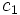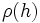# Class-separating field

This term is related to: linear representation theory
View other terms related to linear representation theory | View facts related to linear representation theory

This term associates to every group, a corresponding field property. In other words, given a field, every field either has the property with respect to that group or does not have the property with respect to that group

BEWARE! This term is nonstandard and is being used locally within the wiki. [SHOW MORE]

## Definition

### Symbol-free definition

A field is termed class-separating for a group if it satisfies the following equivalent conditions:

1. Given any two conjugacy classes in the group, there exists a finite-dimensional linear representation of the group over the field such that the images of the conjugacy classes, are not conjugate in the general linear group.
2. Given two elements of the group whose images are conjugate in the general linear group for every finite-dimensional linear representations, the two elements must be conjugate in the group.
3. No two distinct conjugacy classes can be locally conjugate.

### Definition with symbols

A field$k$ is termed class-separating for a group$G$ if it satisfies the following equivalent conditions:

1. Given any two conjugacy classes$c_1$ and$c_2$, there exists a finite-dimensional linear representation$\rho:G \to GL(V)$ where$V$ is a finite-dimensional$k$-vector space, such that$\rho(c_1)$ and$\rho(c_2)$ are not in the same conjugacy class in$GL(V)$.
2. Given two elements$g$ and$h$ in$G$ such that$\rho(g)$ and$\rho(h)$ are conjugate in$GL(V)$ for every finite-dimensional linear representation$\rho$ of$G$, we can conclude that$g$ and$h$ are conjugate elements inside$G$.

### Definition in terms of the conjugacy class-representation duality

Further information: conjugacy class-representation duality

Let$L(c,\rho)$ denote the conjugacy class in$GL(V)$ of the image of the conjugacy class$c$ of$G$ under the representation$\rho$. Then,$k$ is class-separating for$G$ if and only if$L(c_1,\rho) = L(c_2,\rho)$ implies that$c_1 = c_2$.

## Facts

For a finite group, a sufficiently large field is a field of characteristic zero or relatively prime to the order of the group, which contains all the$m^{th}$ roots of unity where$m$ is the exponent of the group.

It turns out that any sufficiently large field is character-separating, and hence also class-separating.